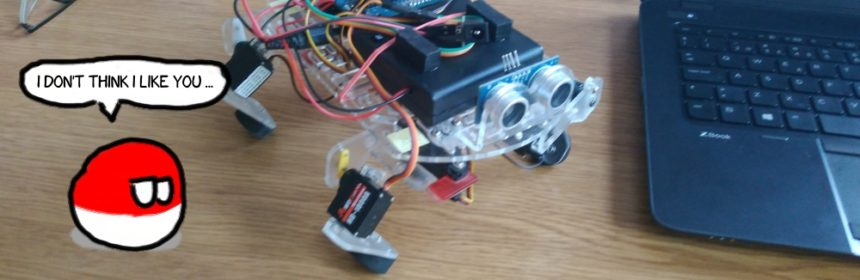# Fixing problems with Matlab + serial connection + fprintf + string variablesSo, last night I had a problem with a simple walking quadruped robot. The whole problem circles the differences between:

```fprintf( robot, '250 250\n')
fprintf( robot, test1)
fprintf( robot, '%s', test1)
```

The robot has a small arduino script that just sends the commands from serial port to PWM driver:

```#include
#include

void setup() {
Serial.begin(57600);
Serial.println("16 channel Servo test!");

pwm.begin();

pwm.setPWMFreq(60);  // Analog servos run at ~60 Hz updates

yield();
}

int leg11 = 250;
int leg12 = 250;
int leg21 = 250;
int leg22 = 250;
int leg31 = 250;
int leg32 = 250;
int leg41 = 250;
int leg42 = 250;

void loop() {
while( Serial.available() > 0 )
{
leg11 = Serial.parseInt();
leg12 = Serial.parseInt();
leg21 = Serial.parseInt();
leg22 = Serial.parseInt();
leg31 = Serial.parseInt();
leg32 = Serial.parseInt();
leg41 = Serial.parseInt();
leg42 = Serial.parseInt();

{
robot();
}
}
}

void robot()
{
pwm.setPWM(0, 0, leg11);
pwm.setPWM(1, 0, leg12);
pwm.setPWM(2, 0, leg21);
pwm.setPWM(3, 0, leg22);
pwm.setPWM(4, 0, leg31);
pwm.setPWM(5, 0, leg32);
pwm.setPWM(6, 0, leg42);
pwm.setPWM(7, 0, leg41);
}
```

Yeah, it’s so primitive that all the syntax-checking-junkies would start screeming and run sideway at the nearest wall while dancing macarena.

Sending the messages works perfectly fine in serial console as long as you stick to the rule: 8 numbers separated by spaces, ended by ‘\n’.
Matlab example works perfectly fine:

```frank = serial( '/dev/ttyUSB4' )
frank.BaudRate = 57600
fopen( frank )
fprintf( frank, '250 250 250 250 250 250 250 250\n' )
```

Even simple loops work:

```while 1
fprintf( frank, '250 250 250 250 250 250 250 250\n' )
pause( 0.2 )
fprintf( frank, '350 250 350 250 350 250 350 250\n' )
pause( 0.2 )
end
```

… but this does NOT:

```while 1
test1 = '250 250 250 250 250 250 250 250\n';
fprintf( frank, test1 )
pause( 0.2 )
test2 = '350 250 350 250 350 250 350 250\n';
fprintf( frank, test2 )
pause( 0.2 )
end
```

Why? It took me a while to realise that… this is NOT the correct syntax for fprintf! Damn you “I spend most of my time in fast prototyping environments and I forgot how to write good code” thingie.
The correct syntax is:

```test2 = '350 250 350 250 350 250 350 250\n';
fprintf( frank, '%s', test2 )
```

because in Matlab if you try to use the short version then those are equivalent (equivalently bad):

```test2 = '350 250 350 250 350 250 350 250\n';
fprintf( frank, test2 )
fprintf( frank, '%s\n', test2 )
```

so yeah – you would end up sending ‘…\n\n’ which you confuse the hell out of arduino.

Story closed. For now.